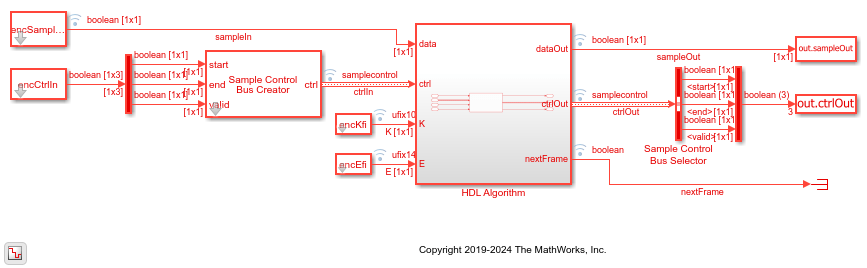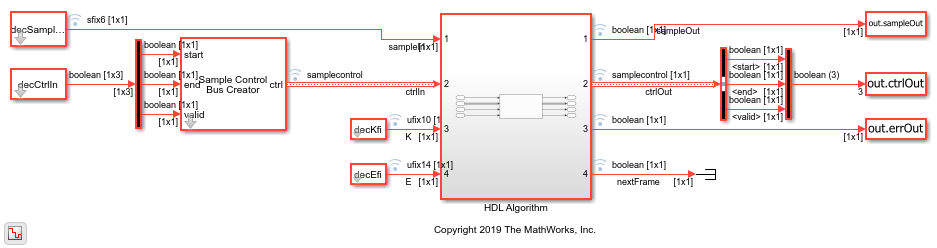# Polar Encode and Decode of Streaming Samples

This example shows how to simulate the NR Polar Encode and Decode blocks and compare the hardware-optimized results with the results from 5G Toolbox™ functions.

### Generate Input Data for Encoder

You must specify the link direction because the coding scheme is different for downlink and uplink messages. Downlink messages are encoded with interleaving and use a CRC length of 24 bits. Uplink messages do not use interleaving, and use a CRC length of 6 (18 < K < 25) or 11 bits (31 < K < 1023).

This example uses uplink mode with K values greater than 31, so each message must have 11 CRC bits.

Choose a series of input values for K and E. These values must be valid pairs supported by the 5G NR standard. Generate random frames of input data and add a CRC codeword.

Convert the message frames to streams of Boolean samples and control signals that indicate the frame boundaries. Generate input vectors of K and E values over time. The example model imports the workspace variables `encSampleIn`, `encCtrlIn`, `encKfi`, `encEfi`, `sampleTime`, and `simTime`.

For this example, the number of invalid cycles between frames is empirically chosen to accommodate the latency of the NR Polar Encoder block for the specified K and E values. When the values of K and E are larger than in this example, the number of invalid cycles between frames must be longer. Use the nextFrame output signal of the block to determine when the block is ready to accept the start of the next input frame.

```K = [132; 132; 132; 54]; E = [256; 256; 256; 124]; numFrames = 4; numCRCBits = 11; idleCyclesBetweenSamples = 0; idleCyclesBetweenFrames = 500; samplesPerCycle = 1; btwSamples = false(idleCyclesBetweenSamples,1); btwFrames = false(idleCyclesBetweenFrames,1); encKfi = []; encEfi = []; dataIn = {numFrames}; for ii = 1:numFrames msg = randi([0 1],K(ii)-numCRCBits,1); msg = nrCRCEncode(msg,'11'); % CRC polynomial is '6' for uplink when 18<K<25, '24C' for downlink encKfi = [encKfi;repmat([fi(K(ii),0,10,0);btwSamples],length(msg),1);btwFrames]; encEfi = [encEfi;repmat([fi(E(ii),0,14,0);btwSamples],length(msg),1);btwFrames]; dataIn{1,ii} = logical(msg); end [encSampleIn,encCtrlIn] = whdlFramesToSamples(... dataIn,idleCyclesBetweenSamples,idleCyclesBetweenFrames,samplesPerCycle); sampleTime = 1; simTime = length(encCtrlIn) + K(numFrames)*2; %#ok<NASGU> ```

### Run Encoder Model

The HDL Algorithm subsystem contains the NR Polar Encoder block. Running the model imports the input signal variables from the workspace and returns a stream of polar-encoded output samples and control signals that indicate the frame boundaries. The NR Polar Encoder block in the model has the Link direction parameter set to `Uplink`, and accepts K and E values from input ports. The model exports variables `sampleOut` and `ctrlOut` to the MATLAB workspace.

```open_system('NRPolarEncodeHDL'); encOut = sim('NRPolarEncodeHDL'); ```### Verify Encoder Results

Convert the streaming data back to frames for comparison with the results of the 5G Toolbox™ `nrPolarEncode` function.

```encHDL = whdlSamplesToFrames(encOut.sampleOut,encOut.ctrlOut); for ii=1:numFrames encRef = nrPolarEncode(double(dataIn{ii}),E(ii),10,false); % last two arguments needed for uplink only error = sum(abs(encRef - encHDL{ii})); fprintf(['Encoded Frame %d: Behavioral and ' ... 'HDL simulation differ by %d bits\n'],ii,error); end ```
```Maximum frame size computed to be 256 samples. Encoded Frame 1: Behavioral and HDL simulation differ by 0 bits Encoded Frame 2: Behavioral and HDL simulation differ by 0 bits Encoded Frame 3: Behavioral and HDL simulation differ by 0 bits Encoded Frame 4: Behavioral and HDL simulation differ by 0 bits ```

### Generate Input Data for Decoder

Use the encoded data to generate input log-likelihood ratios (LLRs) for the NR Polar Decoder block. Use channel, modulator, and demodulator System objects to add noise to the signal.

Again, create vectors of K and E values, and convert the frames of data to streaming samples with control signals. The example model imports the workspace variables `decSampleIn`, `decCtrlIn`, `decKfi`, `decEfi`, `sampleTime`, and `simTime`.

For this example, the number of invalid cycles between frames is empirically chosen to accommodate the latency of the NR Polar Decoder block for the specified K and E values. When the values of K and E are larger than in this example, the number of invalid cycles between frames must be longer. Use the nextFrame output signal of the block to determine when the block is ready to accept the start of the next input frame.

```nVar = 0.7; chan = comm.AWGNChannel('NoiseMethod','Variance','Variance',nVar); bpskMod = comm.BPSKModulator; bpskDemod = comm.BPSKDemodulator('DecisionMethod', ... 'Approximate log-likelihood ratio','Variance',nVar); % more idle cycles greater list lengths. max 5251 for list 4. % 1st pkt LL=8 just over 5000, not sure what is max? % should i make this a more simulink-y example to show how to use the fifo % with the nextframe signal? idleCyclesBetweenFrames = 6000; btwFrames = false(idleCyclesBetweenFrames,1); decKfi = []; decEfi = []; rxLLR = {numFrames}; rxLLRfi = {numFrames}; for ii=1:numFrames mod = bpskMod(double(encHDL{ii})); rSig = chan(mod); rxLLR{1,ii} = bpskDemod(rSig); rxLLRfi{1,ii} = fi(rxLLR{1,ii},1,6,0); decKfi = [decKfi;repmat([fi(K(ii),0,10,0);btwSamples],length(rSig),1);btwFrames]; decEfi = [decEfi;repmat([fi(E(ii),0,14,0);btwSamples],length(rSig),1);btwFrames]; end [decSampleIn,decCtrlIn] = whdlFramesToSamples(... rxLLRfi,idleCyclesBetweenSamples,idleCyclesBetweenFrames,samplesPerCycle); simTime = length(decCtrlIn) + K(numFrames)*2; ```

### Run Decoder Model

The HDL Algorithm subsystem contains the NR Polar Decoder block configured to use a list length of eight. The block in the model also has the Link direction parameter set to `Uplink`, and accepts K and E values from input ports. Running the model imports the input signal variables from the workspace and returns a stream of decoded output samples and control signals that indicate the frame boundaries. The model exports variables `sampleOut`, `ctrlOut`, and `errOut` to the MATLAB workspace. Select the valid values of the `errOut` signal by using the `ctrlOut.valid` signal.

```open_system('NRPolarDecodeHDL'); decOut = sim('NRPolarDecodeHDL'); ```### Verify Decoder Results

Convert the streaming samples returned from the Simulink model into frames for comparison with the results of the 5G Toolbox™ `nrPolarDecode` function.

The `nrPolarDecode` function returns the decoded message, including 24 recalculated CRC bits. The NR Polar Decoder block returns the decoded message without the CRC bits, and returns the CRC status separately on the err port.

The block and function output bits can differ for frames that report a decoding error. The block can return a decoding error in cases when the function successfully decodes the message. The overall decoding performance of the block is very close to that of the function.

```decHDL = whdlSamplesToFrames(decOut.sampleOut,decOut.ctrlOut); errHDL = decOut.errOut(decOut.ctrlOut(:,2)); L = 8; for ii = 1:numFrames decRef = nrPolarDecode(rxLLR{1,ii},K(ii),E(ii),L,10,false,numCRCBits); % last three arguments needed for uplink only [decRef,errRef] = nrCRCDecode(decRef,'11'); % CRC polynomial is '6' for uplink when 18<K<25, '24C' for downlink error = sum(abs(decRef - decHDL{1,ii})); fprintf(['Decoded Frame %d: Behavioral and ' ... 'HDL simulation differ by %d bits\n'],ii,error); msg = dataIn{1,ii}(1:(length(dataIn{ii})-numCRCBits)); loopErr = sum(abs(msg - decHDL{1,ii})); fprintf(['The decoded output message from the HDL simulation',... ' differs from the input message by %d bits \n'],loopErr); errRef = any(errRef); if ~errHDL(ii) && ~errRef fprintf('HDL and behavioral simulations successfully decoded the message. \n'); elseif errHDL(ii) && ~errRef fprintf(['Behavioral simulation successfully decoded the message,',... ' but HDL sim reported a decode error\n']); elseif ~errHDL(ii) && errRef fprintf(['HDL simulation successfully decoded the message,',... ' but behavioral simulation reported a decode error\n']); else fprintf('HDL and behavioral simulations both reported a decode error. \n'); end end ```
```Maximum frame size computed to be 121 samples. Decoded Frame 1: Behavioral and HDL simulation differ by 0 bits The decoded output message from the HDL simulation differs from the input message by 0 bits HDL and behavioral simulations successfully decoded the message. Decoded Frame 2: Behavioral and HDL simulation differ by 0 bits The decoded output message from the HDL simulation differs from the input message by 0 bits HDL and behavioral simulations successfully decoded the message. Decoded Frame 3: Behavioral and HDL simulation differ by 0 bits The decoded output message from the HDL simulation differs from the input message by 0 bits HDL and behavioral simulations successfully decoded the message. Decoded Frame 4: Behavioral and HDL simulation differ by 0 bits The decoded output message from the HDL simulation differs from the input message by 0 bits HDL and behavioral simulations successfully decoded the message. ```